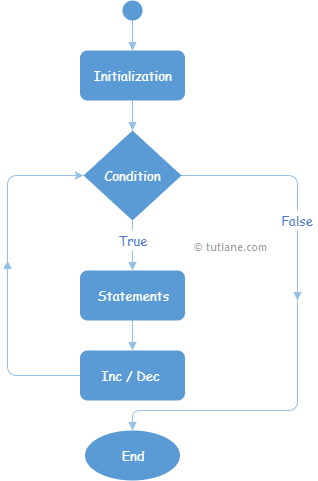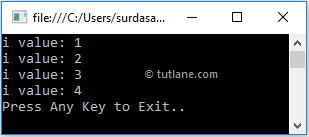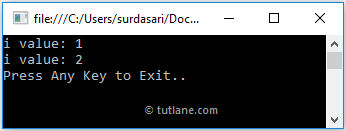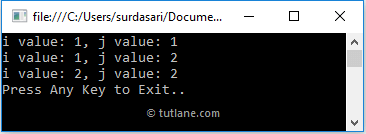# Visual Basic (VB) For Loop

In Visual Basic, For loop is useful to execute a statement or a group of statements repeatedly until the defined condition returns true.

Generally, For loop is useful in Visual Basic applications to iterate and execute a certain block of statements repeatedly until the specified number of times.

## Visual Basic For Loop Syntax

Following is the syntax of defining the `For` loop in Visual Basic programming language.

For variable As [Data Type] = start To end
// Statements to Execute
Next

If you observe the above syntax, we defined `For` loop with different parameters. Here, the variable parameter is require in the `For` statement, and it must be numeric. The Data Type is optional, and it is useful to define the data type for the variable. The start and end parameters are required to define the initial and final value of a variable.

## Visual Basic For Loop Flowchart Diagram

Following is the pictorial representation of `For` loop process flow diagram in Visual Basic programming language.Now, we will see how to use For loop in Visual Basic programming language with examples.

## Visual Basic For Loop Example

Following is the example of using `For` loop in Visual Basic programming language to iterate or loop through a particular list of statements.

Module Module1
Sub Main()
For i As Integer = 1 To 4
Console.WriteLine("i value: {0}", i)
Next
Console.WriteLine("Press Enter Key to Exit..")
End Sub
End Module

If you observe the above code, we defined a `For` loop to iterate over 4 times to print the value of variable i and following are the main parts of For loop.

When we execute the above Visual Basic program, we will get the result as shown below.If you observe the above result, `For` loop was executed four times and printed the variable i value four times.

## Visual Basic For Loop with Exit Statement

In Visual Basic, using the Exit keyword, we can stop the execution of the For loop statement based on our requirements.

Following is the example of stopping the execution of `For` loop using `Exit` statement.

Module Module1
Sub Main()
For i As Integer = 1 To 4
If i = 3 Then Exit For
Console.WriteLine("i value: {0}", i)
Next
Console.WriteLine("Press Enter Key to Exit..")
End Sub
End Module

If you observe the above code, we used `Exit` statement to exit from `For` loop whenever the variable i value equals to 3.

When we execute the above Visual Basic program, we will get the result as shown below.If you observe the above result, whenever the variable i value equals 3, the For loop execution automatically stops.

This is how we can use `Exit` statement in `For` loop to terminate the execution of `For` loop based on our requirements.

## Visual Basic Nested For Loop

In visual basic, we can create one `For` loop within another `For` loop based on our requirements. Following is the example of creating a nested For loop in Visual Basic.

Module Module1
Sub Main()
For i As Integer = 1 To 4
For j As Integer = i To 3 - 1
Console.WriteLine("i value: {0}, j value: {1}", i, j)
Next
Next
Console.WriteLine("Press Enter Key to Exit..")
End Sub
End Module

If you observe the above example, we created a `For` loop within another loop and printing the values based on our requirements.

When we execute the above Visual Basic program, we will get the result as shown below.This is how we can create the nested `For` loops in our Visual Basic programming language based on our requirements.